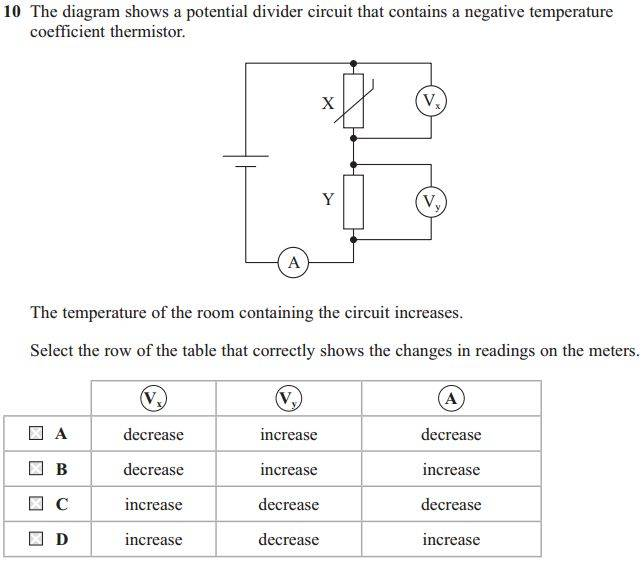# Circuit with NTC/Voltmeter/Ammeter

ravsterphysics

## Homework Statement## The Attempt at a Solution

Since resistance of the NTC falls we can say that the total resistance of the circuit has decreased so there is now more current in the circuit, so it's definitely answer B or D.

And since X's resistance has fallen, PD across X will decrease too (because even though current has increased, the drop in resistance is bigger than the increase in current??)

And since there is more current in the circuit, the pd of Y will increase??

Homework Helper
Gold Member

## Homework Statement

View attachment 111592

## The Attempt at a Solution

Since resistance of the NTC falls we can say that the total resistance of the circuit has decreased so there is now more current in the circuit, so it's definitely answer B or D.

And since X's resistance has fallen, PD across X will decrease too (because even though current has increased, the drop in resistance is bigger than the increase in current??)

And since there is more current in the circuit, the pd of Y will increase??
Right.

•ravsterphysics